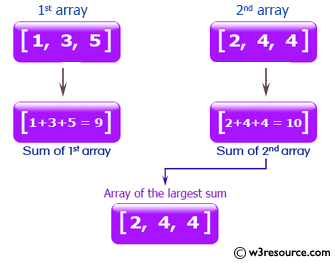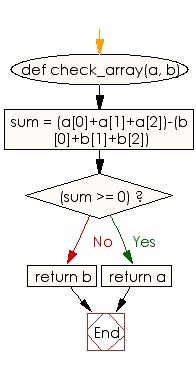﻿ Ruby Array exercises: Compute the sum of two arrays and return the array which has the largest sum - w3resource# Ruby Array Exercises: Compute the sum of two arrays and return the array which has the largest sum

## Ruby Array: Exercise-21 with Solution

Write a Ruby program to compute the sum of two arrays (length 3) and return the array which has the largest sum.Ruby Code:

``````def check_array(a, b)
sum = (a+a+a)-(b+b+b)
if(sum >= 0)
return a
end
return b
end
print check_array([1, 3, 5], [2, 4, 4]),"\n"
print check_array([11, 3, 5], [21, 0, -4]),"\n"
``````

Output:

```[2, 4, 4]
[11, 3, 5]
```

Flowchart:Ruby Code Editor: